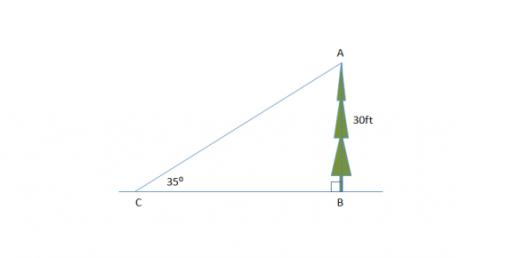# Quiz About Similarity & Congruency

8 Questions | Attempts: 673
ShareSettingsA quiz to test your understanding about Similarity & Congruency in Geometry. Two objects are similar if they have the same shape, so that one is an enlargement of the other. Two objects are congruent if they are the same shape and size. Best of luck for the quiz!

• 1.
In the following triangles, which are congruent?
• A.

(1) and (2) only

• B.

(1) and (3) only

• C.

(2) and (3) only

• D.

All of them

• E.

None of them

• 2.
A circular cone of height h units is place with its base on table. It contains water to a depth of 2/3 h. What is the ratio of the volume of water to that of the cone?
• A.

1:27

• B.

2:3

• C.

8:27

• D.

19:27

• E.

26:27

• 3.
A trapizium ABCD with sides AB // DC. Diagonals AC and BD meet at point K. Which of the following statements is/are correct?(1) triangle AKD and triangle BKC have the same area.(2) triangle AKB and triangle CKD are similar.(3) angle DAK = angle CBK
• A.

(1) only

• B.

(2) only

• C.

(3) only

• D.

(1) and (2) only

• E.

(2) and (3) only

• 4.
Which of the following cases can represent a pair of congruent triangles?
• A.

(1) only

• B.

(2) only

• C.

(3) only

• D.

(1) and (2) only

• E.

(1) and (3) only

• 5.
ADE is a triangle. Points B and C divides the side AD in the ratio AB:BC:CD=1:2:3. Points G and F are on the side AE such that AG:GF:FE=1:2:3. If BG//CF//DE. What is the ratio of the area of the trapezium BGCF to the area of triangle ADE?
• A.

1:2

• B.

1:3

• C.

3:8

• D.

2:9

• E.

4:9

• 6.
A right circular cone is placed with its base on the table. The cone is divided into 3 portions by planes parallel to the base. The height of each portion is h units and the portions are named A, B and C, A being the top most portion. The base radius of A is r units. What is the ratio(express answer in the form x:y, no spacing in between) of the volume of A to that of B?
• 7.
A right circular cone is placed with its base on the table. The cone is divided into 3 portions by planes parallel to the base. The height of each portion is h units and the portions are named A, B and C, A being the top most portion. The base radius of A is r units. What is the ratio(express answer in the form x:y, no spacing in between) of the volume of B to that of C?
• 8.
A right circular cone is placed with its base on the table. The cone is divided into 3 portions by planes parallel to the base. The height of each portion is h units and the portions are named A, B and C, A being the top most portion. The base radius of A is r units. What is the ratio(express answer in the form x:y, no spacing in between) of the surface area of B to that of C?

## Related TopicsBack to top
×

Wait!
Here's an interesting quiz for you.